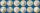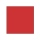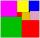# Garden

Area of a square garden is 6/4 of triangle garden with sides 56 m, 35 m, and 35 m.

How many meters of fencing need to fence a square garden?

Result

x =  119 m

#### Solution:

$S_{ \square} = \dfrac{ 6 } 4 S_{\triangle} \ \\ a^2 = \dfrac{ 6 } 4 \cdot \dfrac{ 1 } {2 } \cdot 56 \cdot \sqrt{ 35^2- \dfrac{ 56^2 } { 4 } } = 882 \ m^2 \ \\ a = 29.7 \ m \ \\ S_{ \square} = 882 \ m^2 \ \\ S_{ \triangle} = 588 \ m^2 \ \\ \ \\ x = 4a = 119 \ \text { m }$

Our examples were largely sent or created by pupils and students themselves. Therefore, we would be pleased if you could send us any errors you found, spelling mistakes, or rephasing the example. Thank you!

Leave us a comment of this math problem and its solution (i.e. if it is still somewhat unclear...):Be the first to comment!#### Following knowledge from mathematics are needed to solve this word math problem:

Do you have a linear equation or system of equations and looking for its solution? Or do you have quadratic equation? Pythagorean theorem is the base for the right triangle calculator. Do you want to convert length units? See also our trigonometric triangle calculator.

## Next similar math problems:

1. GardensThe garden has the square shape with circumference 124 m. Divide it into two rectangular gardens, one should circumference 10 meters more than the second. What size will have a gardens?
2. Rectangle poolDetermine dimensions of open pool with a square bottom with a capacity 32 m3 to have painted/bricked walls with least amount of material.
3. Cuboid and eq2Calculate the volume of cuboid with square base and height 6 cm if the surface area is 48 cm2.
4. A photographA photograph will stick to a white square letter with a x cm length. The photo is 3/4 x cm long and 20 cm wide than the width of the paper. The surface of the remaining paper surrounding the photograph is 990 cm2. Find the size of paper and photo.
5. Two squaresTwo squares whose sides are in the ratio 5:2 have sum of its perimeters 73 cm. Calculate the sum of area this two squares.
6. SquareIf the length of the sides of the square we decrease by 25% decrease the content area of 28 cm2. Determine the side length of the original square.
7. Rectangle vs squareOne side of the rectangle is 1 cm shorter than the side of the square, the second side is 3 cm longer than the side of the square. Square and rectangle have the same content. Calculate the length of the sides of a square and a rectangle.
8. CoinsHarvey had saved up a number of 2-euro coins. He stored coins in a single layer in a square. Left 6 coins. When he make square, which has one more row, missing 35 coins. How many euros he have?
9. Three shapes1/5 of a circle is shaded. The ratio of area if square to the sum of area of rectangle and that of the circle is 1:2. 60% of the square is shaded and 1/3 of the rectangle is shaded. What is the ratio of the area of circle to that of the rectangle?
10. Square vs rectangleSquare and rectangle have the same area contents. The length of the rectangle is 9 greater and width 6 less than side of the square. Calculate the side of a square.
11. Square and rectangleCalculate the side of a square which content area equals area of the rectangle having a length of 3 cm greater and by 2 cm smaller than the side of the square.
12. Unknown number 24I think the number: a - is the same as the square area that has the 12th circumfence. What is this number? b - its half is 7 times bigger than its quarter. Is this the number?
13. Equilateral triangleA square is inscribed into an equilateral triangle with a side of 10 cm. Calculate the length of the square side.
14. MO-Z5-3-66 tilesThe picture shows a square tiles with side 10 dm which is composed of four identical small rectangles and squares. Circumference of small square is five times smaller than the circumference of the entire tile. Determine the dimensions of the rectangle.
15. Square sidesIf the square side increases by 28%, the square circumference increases by 11.2 meters. Find the length of the original square side.
16. SquareIf we increase one side of square by its one-half then square perimeter increase by 10 cm. What is the side of the square?
17. Brick weightThe brick weighs 2 kg and a half bricks. How much does one brick weigh?